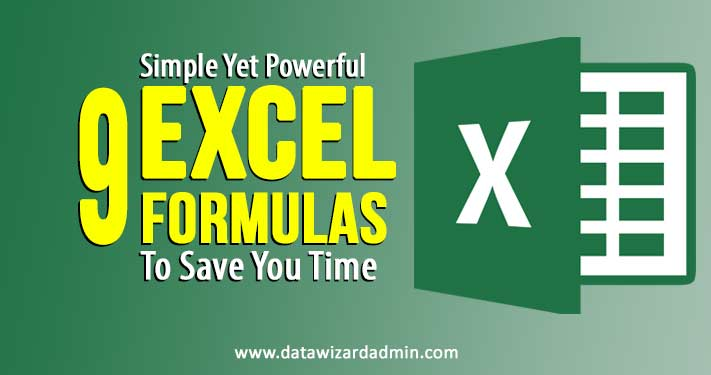# 9 Simple Yet Powerful Excel Formulas To Save You Time

Do you use Excel formulas in your calculations? In this article, we explore 9 simple, yet powerful excel formulas that will save you time when analysing your accounts spreadsheets and reduce the risk of human error.

Many of the examples I will share with you are very handy when it comes to keeping your accounts in order. I am not saying you can fire your Accountant, far from it, but they will help you to keep on top of your records and see how your finances are performing.

Simple Multiplication, Division, Subtraction & Addition

Where is a calculator when you need one? With excel you can carry out simple equations by hitting just a few buttons too! In the example below, you can see that there is an overall cost of a purchase. If you wanted to calculate the individual price, you can perform a simple division (/) formula and get the individual price. To do this, click on the cell where you want to display the answer and select = then click on the cell that shows the overall price, type / and then click on the cell that shows the number of items. In this example the formula shows as =C4/D4 – C4 is the cell number of the overall cost and D4 is the cell showing the number of items. When you hit enter your answer will be displayed.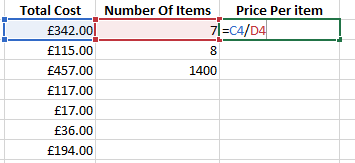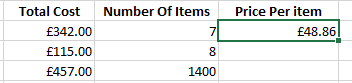The same can be applied in reverse if you have the individual price and the number of items and wanted to know the overall cost. In this instance, you would use the * as a multiplication symbol and the formula would look something like this: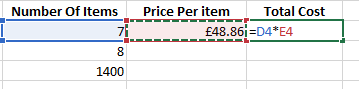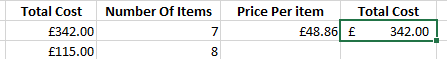As you can see, again you would start with the equals sign = then click on the first cell displaying the number of tems, type * and then click on the price per item cell and hit enter. So you formula would be =D4*E4. This is especially handy if you want to calculate the amount of VAT on an item. To do this, you would follow the instructions above, but instead of clicking on the second cell you would simply type *0.2. So it would look something like this:-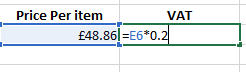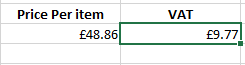Addition and subtraction are performed exactly the same way as the division and multiplication formulas.

=SUM()

What if you wanted to add more than just the totals from 2 cells? This is where the =SUM() formula comes in.

This can be done two ways. The first is to select the cell you want the answer to be displayed and type =SUM( then type the first cell number followed by : and the second cell number then ). A quicker way, however is to type =SUM( then click on the first cell and hold the left mouse click down and drag across all cells to be included in the equation, then release and type ) then hit return. The formula will look something like this:

As you can see, the formula in this example is =SUM(C4:C11) and the result is the total of all those cells added together. This is a great formula to use when you want to add up all expenses or invoices in a particular period.

=AVERAGE

When looking at your monthly expenditure over a longer period you may find that your income fluctuates quite a bit depending on how much business you are generating and you want to know what your average monthly revenue is. This is where the =AVERAGE() formula works well.

It does exactly what it says and calculates the average of the cells within the equation.

In this example we wanted to find out the average gross monthly income from our Profit and Loss sheets. To do this, as always, click on the cell where you want to display the results and type =AVERAGE( then select the relevant cells to include either by using your mouse to click and drag or holding the Ctrl key and clicking on specific cells if they are not all together, then end the formula with ) and hit enter.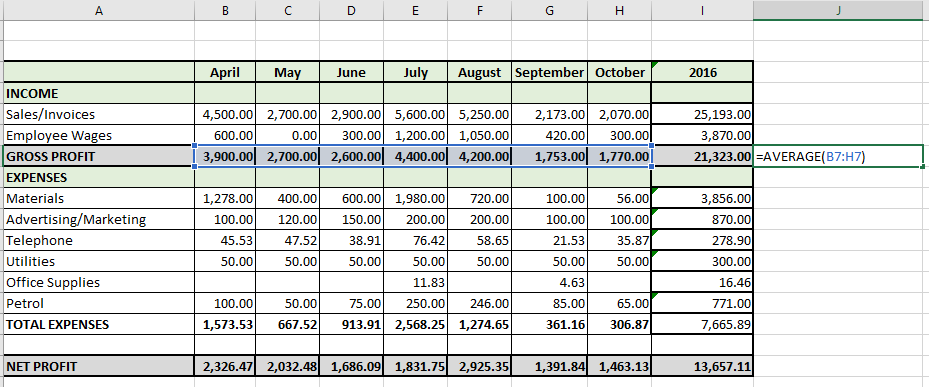At first glance, it would be impossible to work out what your average gross profit is in this spreadsheet without getting your calculator out and adding all the individual cells then dividing by the number of cells in total. However, with the =AVERAGE() formula, it has calculated it easily and below shows you the result.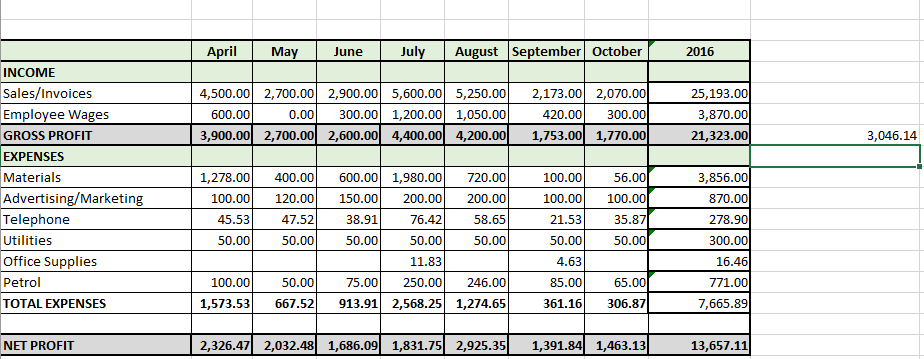=NOW()

Sometimes it is handy to have the current date showing on a spreadsheet so you know you are working on a current document. This is one of the simplest formulas. Just click on the cell where you want the date to appear and type =NOW() and magically the date will appear. This often shows the full date and current time. However, if you only want the date to show, you can right click on the cell, select Format Cells, Select the Number tab and choose Date and the way you want the date to be displayed. This is very handy when we come to the next formula I am going to share with you.

=DAYS()

Want to know the number of days between two dates in a spreadsheet? Maybe you want to see how long ago you sent an invoice to a customer so that you know when to chase them up for payment. This is where the =DAYS() formula becomes very handy, especially when used in conjunction with the =NOW() formula, means that whenever you open the spreadsheet you can see an up-to-date analysis of overdue payments.

To use this, click on the relevant cell to display the number and type =DAY( then select the cell that is displaying the CURRENT or LATEST date (When using the =NOW formula you select the cell this is displayed in). Then type a comma , and click on the cell that shows the first date i.e. the invoice date and close the formula off with a bracket ) and hit enter.

Now you can clearly see how many days ago you sent the invoice and whether it is time to chase the customer for payment. Using the =NOW() formula also means that the next time you go into the spreadsheet, the number of days outstanding will be automatically updated.

=MIN()

There may be times when you are looking through a very large spreadsheet and need to find the smallest number in a range of cells, maybe you want to see your lowest income achieved since you started. This is where the =MIN() formula can help.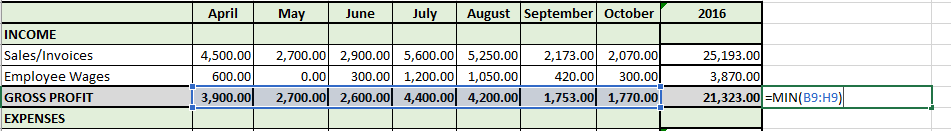In this example, it is quick to see. However, if you have been in business for some time, this could take you ages. To find the result, click on the cell that you want the answer to be displayed and type =MIN( then select the relevant cells you want to analyse in the usual way, type ), hit enter, and the result will be revealed.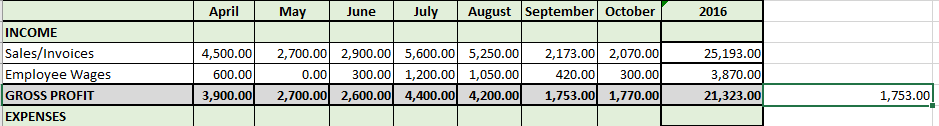=MAX()

Alternatively, you may want to find out what the largest number in a set of cells is. You follow the same process as for the =MIN() formula, except replace the MIN with MAX.

=COUNT()

What if you want to see how many invoices you have sent in a period? Or how many months you have been trading. The =COUNT() formula can do this in an instant. This is NOT a formula to add the total value of the invoices, for that you would you the =SUM() formula. The =COUNT() formula adds how many cells contain a number. Here is how you do it. Once again, select the cell where the results are to be displayed and type =COUNT( then select the relevant cells. Don’t worry about any cells that contain text, the formula will ignore them, then close the bracket ) and hit enter.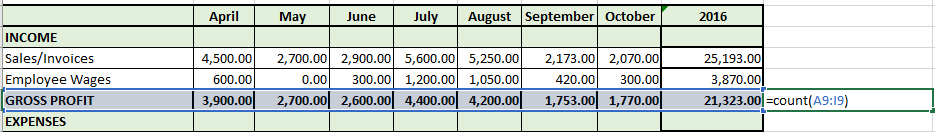As you can see below. Field A8 (GROSS PROFIT) has been ignored and only the cells containing a number have been counted.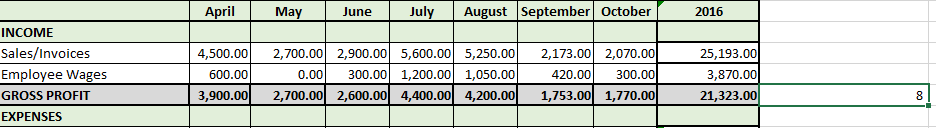Copy Results From Another Sheet

We don’t always hold all of the data needed on a single sheet, so there are times when we need to gather relevant information from another tab or completely different spreadsheet. It may be that you have the cost of your materials broken down on one page and want to add the total expenditure to your Profit and Loss accounts for the month. Doing this manually can leave you open to error. However, a simple formula can help you here. To do this, ensure that all spreadsheets needed are open.

In this example, we have a separate tab where the cost of materials and date purchased are listed. We have used the simple =SUM formula to add the total cost of the materials for the month of April and now want to automatically populate the Profit and Loss tab for the same period.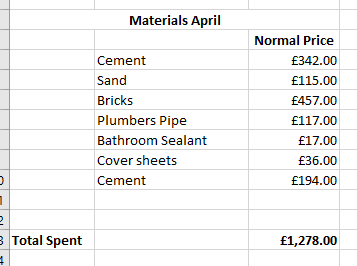As we want the total to show in the cell marked ‘Materials’ for the month of April, you simply click on that cell, type = then move to the tab or spreadsheet where you want to acquire the information from, in this case it would be the cell that has the total cost value, then hit return.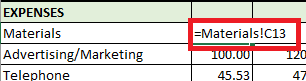As you can see above, it clearly displays the name of the tab or spreadsheet (Materials!) and the cell number and that’s how easily you can merge your data from one tab/spreadsheet to another.

So there you have it. I’ve now shown you 9 simple, yet powerful, excel formulas to save you valuable time when analysing or updating your accounts. If you are ever unsure whether you have selected the right cells, just check the outline colours as each part of the formula is colour coded with the relevant cells highlighted in the same colour.

What simple formulas have you found that can help you? We would love to hear in the comments below.

Do you need formulas set up on your spreadsheets but not sure where to start? Then get in contact. Call Lyn on 01329 481202 or click on the Request Call Back button below and we would be happy to chat about how we can help.